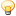CodeGuru Home VC++ / MFC / C++ .NET / C# Visual Basic VB Forums Developer.com

# Thread: two dimensional array explanation

1.Junior MemberJoin Date
Jan 2018
Location
Java 8
Posts
16

##two dimensional array explanation

Hey guys, was working one this practice problem and was able to solve it but was looking at two other solutions that seemed more simple but I don't completely understand them. was wondering if someone could explain them to me a little more.

This is the practice problem:
Code:
```After they became famous, the CodeBots all decided to move to a new building and live together. The building is represented by a rectangular matrix of rooms. Each cell in the matrix contains an integer that represents the price of the room. Some rooms are free (their cost is 0), but that's probably because they are haunted, so all the bots are afraid of them. That is why any room that is free or is located anywhere below a free room in the same column is not considered suitable for the bots to live in.

Help the bots calculate the total price of all the rooms that are suitable for them.

Example

For
matrix = [[0, 1, 1, 2],
[0, 5, 0, 0],
[2, 0, 3, 3]]
the output should be
matrixElementsSum(matrix) = 9.

Here's the rooms matrix with unsuitable rooms marked with 'x':

[[x, 1, 1, 2],
[x, 5, x, x],
[x, x, x, x]]
Thus, the answer is 1 + 5 + 1 + 2 = 9.

For
matrix = [[1, 1, 1, 0],
[0, 5, 0, 1],
[2, 1, 3, 10]]
the output should be
matrixElementsSum(matrix) = 9.

Here's the rooms matrix with unsuitable rooms marked with 'x':

[[1, 1, 1, x],
[x, 5, x, x],
[x, 1, x, x]]
Note that the free room in the first row make the full column unsuitable for bots.

Thus, the answer is 1 + 1 + 1 + 5 + 1 = 9.```
This is my solution, where I leave the zero's as is and change the ones below them to zero's as well, then I add all the remaining untouched numbers:

Code:
```int matrixElementsSum(int[][] matrix) {
int suitableTotal =0;

for(int row=0; row<matrix.length; row++){
for(int col=0; col<matrix[row].length; col++){
if(matrix[row][col] == 0){
for(int i = row+1; i<matrix.length; i++){
matrix[i][col] = 0;
}
}
}
}

for(int row=0; row<matrix.length; row++){
for(int col=0; col<matrix[row].length; col++){
if(matrix[row][col] != 0)
suitableTotal += matrix[row][col];
}
}

return suitableTotal;```
Then there was this solution where the boolean array confused me:
Code:
```int matrixElementsSum(int[][] matrix) {
boolean[] array = new boolean[matrix.length];
int sum = 0;

for (int i = 0; i < matrix.length; i++) {
for (int j = 0; j < matrix[i].length; j++) {
if (! array[j]) {
if (matrix[i][j] != 0)
sum += matrix[i][j];
else
array[j] = true;
}
}
}

return sum;```
and the second solution I found, to my understanding it has a for loop for the column and then a nest for loop for the rows. The if statement is confusing me a little. because at row 2, column 0 the value is 2 which is > 0 but the 2 is below the zero. Im trying to figure out how this whole code determines that even if its greater then 0 it below a zero from the previous row.

Code:
```int matrixElementsSum(int[][] matrix) {
int sum = 0;
for(int i =0; i < matrix.length; i++) {
for(int j=0; j < matrix.length; j++) {
if( matrix[j][i] > 0 )
sum +=matrix[j][i];
else
break;
}
}
return sum;
}

// [0,1,1,2]
// [0,5,0,0]
// [2,0,3,3]```Reply With Quote

2. ## Re: two dimensional array explanation

Look at the indexes if the array in the if statement. Notice how the i and the j are reversed?Reply With Quote

3.Junior MemberJoin Date
Jan 2018
Location
Java 8
Posts
16

## Re: two dimensional array explanationOriginally Posted by ArjayLook at the indexes if the array in the if statement. Notice how the i and the j are reversed?
thank you, I guess I was thinking about it too hard.Reply With Quote

####Posting Permissions

• You may not post new threads
• You may not post replies
• You may not post attachments
• You may not edit your posts
•Click Here to Expand Forum to Full Width

On-Demand Webinars (sponsored)